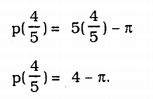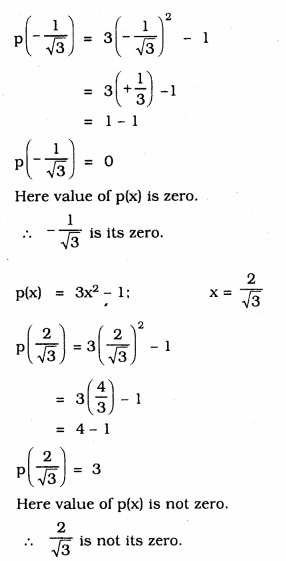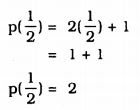# KSEEB Solutions for Class 9 Maths Chapter 4 Polynomials Ex 4.2

KSEEB Solutions for Class 9 Maths Chapter 4 Polynomials Ex 4.2 are part of KSEEB Solutions for Class 9 Maths. Here we have given Karnataka Board Class 9 Maths Chapter 4 Polynomials Exercise 4.2.

## Karnataka Board Class 9 Maths Chapter 4 Polynomials Ex 4.2

Question 1.
Find the value of the polynomial 5x – 4x2 + 3 at
i) x = 0
ii) x = -1
iii) x = 2
i) f(x) = 5x – 4x2 + 3 x = 0 then,
f(0) = 5(0) – 4(0)2 + 3
=0 – 0 + 3
f(0) = 3

ii) f(x) = 5x – 4x2 + 3 x = -1 then,
f(-1) = 5(-1) – 4(-1)2 + 3
= -5 – 4(+1) + 3
= -5 – 4 + 3
= -9 + 3
f(-1) = -6

iii) f(x) = 5x – 4x2 + 3 x = 2 then,
f(2) = 5(2) – 4(2)2 + 3
= 5(2) – 4(4) + 3
= 10 – 16 + 3
= 13 – 16
f(2) = -3

Question 2.
Find p(0), p(1) and p(2) for each of the following polynomials :
i) p(y) = y2 – y + 1
ii) p(t) = 2 + t + 2t2 – t3
iii) p(x) = x3
iv) p(x) = (x – 1) (x + 1)
i) (a) p(y) = y2 – y + 1
p(0) = (0)2 – 0 + 1
= 0 – 0 + 1
∴ p(0) = =1

(b) p(y) = y2 – y + 1
p(1) = (1)2 – 1 + 1
= 1 – 1 + 1
∴ p(1) = 1

(c) p(y) = y2 – y +
p(2) = (2)2 – 2 + 1
= 4 – 2 + 1
= 5 – 2
∴ p(2) = 3

ii) (a) p(t) = 2 + t + 2t2 – t3
p(0) = 2 + 0 + 22 – (0)3
= 2 + 0 + 0 – 0
∴ p(0) = 2

(b) p(t) = 2 + t + 2t2 – t3
p(1) = 2 + 1 + 2(1)2 – (1)3
= 2 + 1 + 2(1) – 1
= 2 + 1 + 2 – 1
∴ p(1) = 4

(c) p(t) – 2 + t + 2t2 – t3
p(2) = 2 + 2 + 2(2)2 – (2)3
= 2 + 2 + 2(4) – 8
= 2 + 2 + 8 – 8
∴ p(2) = 4

iii) (a) p(x) = x3
p(0) = (0)3
p(0) = 0

(b) p(x) = x3
p(1) = (1)3
∴ p(1) = 1

(c) p(x) = x3
p(2) = (2)3
∴ p(2) = 8

iv) (a) p(x) = (x – 1)(x + 1)
p(x) = x2 – 1 [∵ (a + b)(a – b) = a2 – b]
p(0) = (0)2 – 1
= 0 – 1
∴ p(o) = -1

(b) p(x) = (x – 1)(x + 1)
p(x) = x2 – a [∵ (a + b)(a – b) = a2 – b2]
p(1) = (1)2 – 1
= 1 – 1
∴ p(1) = 0

(c) p(x) = (x – 1)(x + 1)
p(x) = x2 – 1 [∵ (a + b)(a – b) = a2 – b2]
p(2) = (2)2 – 1
= 4 – 1
∴ p(0) = 3

Question 3.
Verify whether the following are zeroes of the polynomial, induced against them.
i) p(x) = 3x + 1; $$x=-\frac{1}{3}$$
ii) p(x) = 5x – π; $$x=\frac{4}{5}$$
iii) p(x) = x2 – 1; x = 1, -1
iv) p(x) = (x + 1) (x – 2); x = -1, 2
v) p(x) = x2; x = 0
vi) p(x) = lx + m; $$x=-\frac{m}{l}$$
vii) p(x) = 3x2 – 1; $$x=-\frac{1}{\sqrt{3}}, \frac{2}{\sqrt{3}}$$
viii) p(x) = 2x + 1; $$x=\frac{1}{2}$$
i) p(x) = 3x + 1; $$x=-\frac{1}{3}$$Here value of polynomial is zero.
$$x=-\frac{1}{3}$$ is not zero of the polynomial

ii) p(x) = 5x – π; $$x=\frac{4}{5}$$Here value of polynomial is not zero.
$$x=\frac{4}{5}$$ is not zero of the polynomial

iii) p(x) = x2 – 1; x = 1, -1
p(1) = (1)2 – 1
= 1 – 1
p(1) = 0
Here value of p(x) is zero.
hence its zero is 1.
p(x) = x2 – 1; x = -1
p(-1) = (-1)2 – 1
= 1 – 1
p(-1) = 0
Here value of p(x) is zero.
∴ -1 is zero.

iv) p(x) = (x – 1)(x – 2); x = -1, 2
p(x) = x2 – 2x + x – 2
p(x) = x2 – x + 2 x = -1
p(-1) = (-1)2 – (-1)2 + 2
= 1 + 1 + 2
p(-1) = 4
Here value of polynomila is not zero.
∴ -1 is not zero.
p(x) = x2 – x + 2 x = 2
p(2) = (2)2 – (2) + 2
= 4 – 2 + 2
p(-1) = 4
Here value of polynomial is not zero.
∴ 2 is not zero.

(v) p(x) = x2; x = 0
p(0) = (0)2
p(0) = 0
Here value of p(x) is zero.
∴ 0 is its zero.

vi) p(x) = lx + m; $$x=-\frac{m}{l}$$
$$\mathrm{p}\left(-\frac{\mathrm{m}}{l}\right)=l\left(-\frac{\mathrm{m}}{l}\right)+\mathrm{m}$$
= -m + m
= 0
Here p(x) is zero.
∴ $$-\frac{\mathrm{m}}{l}$$ is its zero.

(vii) p(x) = 3x2 – 1; $$x=-\frac{1}{\sqrt{3}}, \frac{2}{\sqrt{3}}$$viii) p(x) = 2x + 1; $$x=\frac{1}{2}$$Here value of p(x) is not zero.
∴ $$\frac{1}{2}$$ is not its zero.

Question 4.
Find the zero of the polynomial in each of the following cases :
(i) p(x) = x + 5
(ii) p(x) = x – 5
(iii) p(x) = 2x + 5
(iv) p(x) = 3x – 2
(v) p(x) = 3x
(vi) p(x) = ax, a ≠ 0
(vii) p(x) = cx + d, c ≠ 0, c, d are real numbers.
i) p(x) = x + 5
Let p(x) =0, then,
p(x) = x + 5 = 0
x = 0 – 5
∴ x = -5
-5 is zero of p(x).

ii) p(x) = x – 5
If p(x) = 0, then
p(x) = x – 5 = 0
x = 0 + 5
∴ x = 5
5 is the zero of p(x).

iii) p(x) = 2x + 5
If p(x)= 0, then
p(x) = 2x + 5 = 0
2x = – 5
∴ $$x=\frac{-5}{2}$$
$$\frac{-5}{2}$$ is the zero of p(x).

iv) p(x) = 3x – 2
If p(x)= 0, then
p(x) = 3x – 2 = 0
3x = 2
∴ $$x=\frac{2}{3}$$
$$\frac{2}{3}$$ is the zero of p(x).

v) p(x) = 3x
If p(x) = 0, then
p(x) = 3x = 0
∴ $$x=\frac{0}{3}$$
$$\frac{0}{3}$$ is the zero of p(x)

vi) p(x) = ax, a ≠ 0
If p(x)= 0, then
p(x) = ax = 0
∴ $$x=\frac{0}{a}$$ ∴ x = ∞(infinity)
∞ is the zero of p(x).

vii) p(x) = cx + d, c ≠ 0, c, d are real numbers
If p(x)= 0, then
p(x) = cx + d = 0
cx = 0 – d
cx = -d
∴ $$x=-\frac{d}{c}$$
$$-\frac{\mathrm{d}}{\mathrm{c}}$$ is the zero of p(x).

We hope the KSEEB Solutions for Class 9 Maths Chapter 4 Polynomials Ex 4.2 help you. If you have any query regarding Karnataka Board Class 9 Maths Chapter 4 Polynomials Exercise 4.2, drop a comment below and we will get back to you at the earliest.

error: Content is protected !!